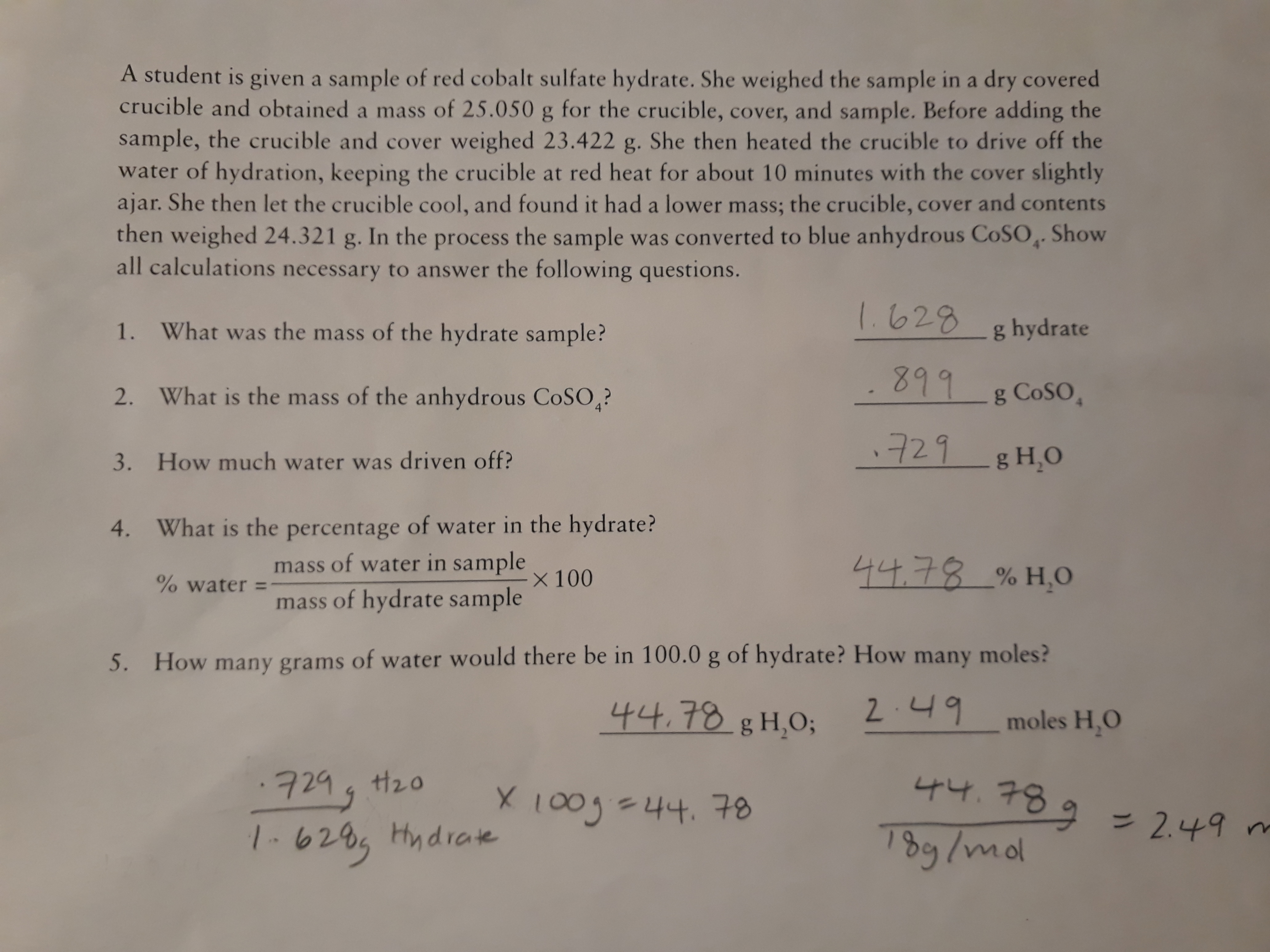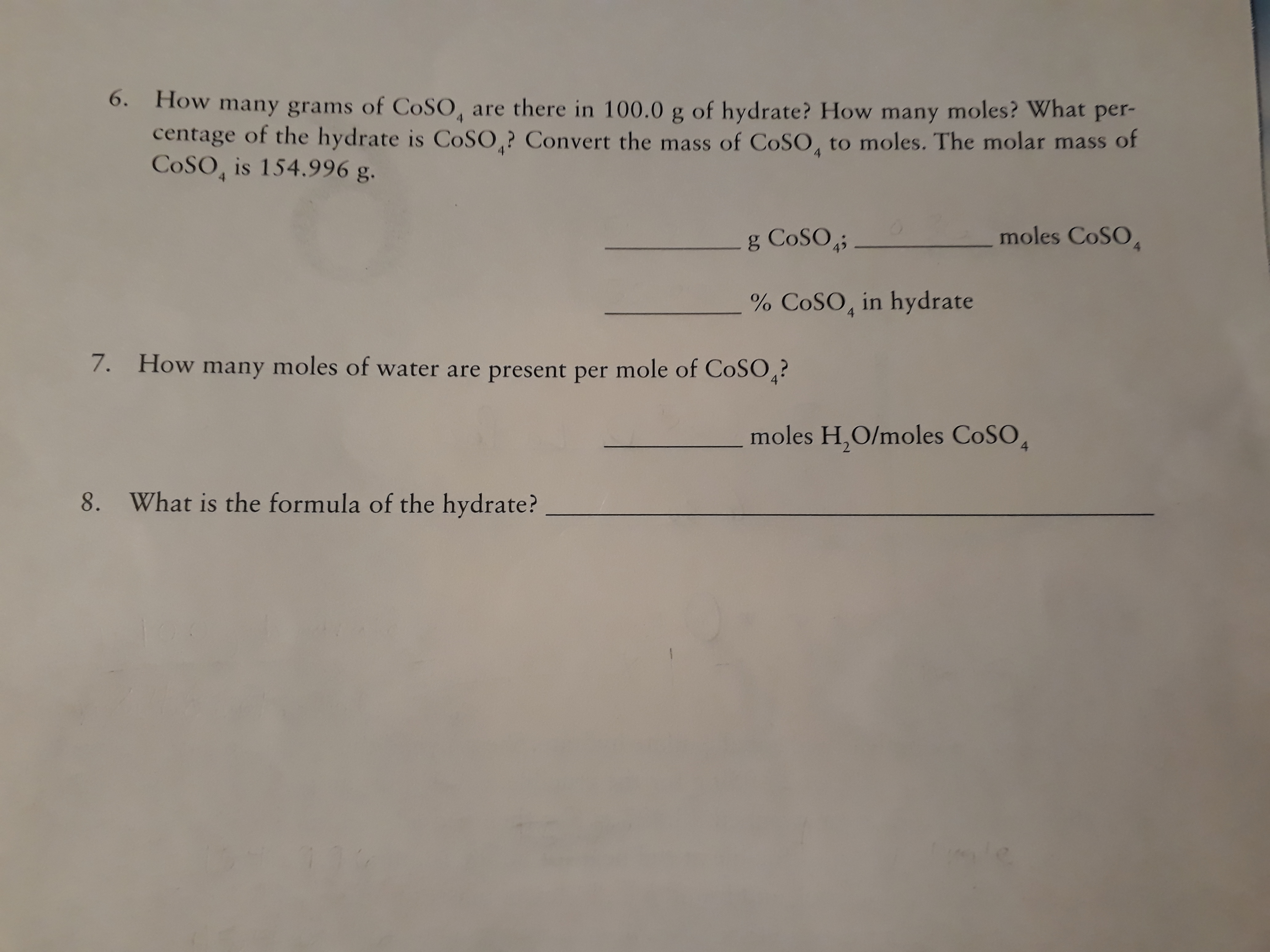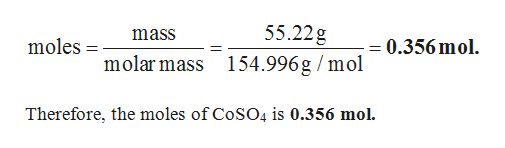# A student is given a sample of red cobalt sulfate hydrate. She weighed the sample in a dry coveredcrucible and obtained a mass of 25.050 g for the crucible, cover, and sample. Before adding thesample, the crucible and cover weighed 23.422 g. She then heated the crucible to drive off thewater of hydration, keeping the crucible at red heat for about 10 minutes with the cover slightlyajar. She then let the crucible cool, and found it had a lower mass; the crucible, cover and contentsthen weighed 24.321 g. In the process the sample was converted to blue anhydrous COSO Showall calculations necessary to answer the following questions.1628g hydrate1.What was the mass of the hydrate sample?899g COSO2.What is the mass of the anhydrous COSO4?721 gHO3.How much water was driven off?4.What is the percentage of water in the hydrate?mass of water in sampleX 100나나,구8 % H,O% watermass of hydrate sample5.How many grams of water would there be in 100.0 g of hydrate? How many moles?나나,78 g H,O;2.니9moles H,O7294 t20X 1003-44.78나나, 구8 92.49 m/-620, HydrateDg/mo 6. How many grams of COSO, are there in 100.0 g of hydrate? How many moles? What per-centage of the hydrate is COSO,? Convert the mass of COSO, to moles. The molar mass ofCoSO, is 154.996 g.444g COSOmoles CoSO% COSO, in hydrate7.How many moles of water are present per mole of COSO?4moles H,O/moles CoSO48.What is the formula of the hydrate?

Question
72 viewshelp_outlineImage TranscriptioncloseA student is given a sample of red cobalt sulfate hydrate. She weighed the sample in a dry covered crucible and obtained a mass of 25.050 g for the crucible, cover, and sample. Before adding the sample, the crucible and cover weighed 23.422 g. She then heated the crucible to drive off the water of hydration, keeping the crucible at red heat for about 10 minutes with the cover slightly ajar. She then let the crucible cool, and found it had a lower mass; the crucible, cover and contents then weighed 24.321 g. In the process the sample was converted to blue anhydrous COSO Show all calculations necessary to answer the following questions. 1628 g hydrate 1. What was the mass of the hydrate sample? 899 g COSO 2. What is the mass of the anhydrous COSO4? 721 gHO 3. How much water was driven off? 4. What is the percentage of water in the hydrate? mass of water in sample X 100 나나,구8 % H,O % water mass of hydrate sample 5. How many grams of water would there be in 100.0 g of hydrate? How many moles? 나나,78 g H,O; 2.니9 moles H,O 7294 t20 X 1003-44.78 나나, 구8 9 2.49 m /-620, Hydrate Dg/mo fullscreenhelp_outlineImage Transcriptionclose6. How many grams of COSO, are there in 100.0 g of hydrate? How many moles? What per- centage of the hydrate is COSO,? Convert the mass of COSO, to moles. The molar mass of CoSO, is 154.996 g. 4 4 4 g COSO moles CoSO % COSO, in hydrate 7. How many moles of water are present per mole of COSO? 4 moles H,O/moles CoSO 4 8. What is the formula of the hydrate? fullscreen
check_circle

star
star
star
star
star
1 Rating
Step 1

Calculation of amount of CoSO4 in 100 g of hydrate:

In 100 g of the hydrate, the amount of water present is 44.78 g. Then, the amount of CoSO4 in 100 g of hydra...help_outlineImage Transcriptionclose55.22g mass = 0.356 mol. moles = molar mass 154.996g /mol Therefore, the moles of CoSO4 is 0.356 mol. fullscreen

### Want to see the full answer?

See Solution

#### Want to see this answer and more?

Solutions are written by subject experts who are available 24/7. Questions are typically answered within 1 hour.*

See Solution
*Response times may vary by subject and question.
Tagged in

### Chemistry